## Example Questions

### Example Question #1 : How To Use Scientific Notation

The speed of light is approximately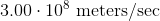.

In scientific notation how many kilometers per hour is the speed of light?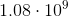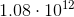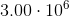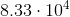Explanation:

For this problem we need to convert meters into kilometers and seconds into hours. Therefore we get,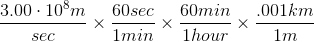Multiplying this out we get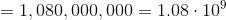### Example Question #11 : Gre Quantitative Reasoning

If one mile is equal to 5,280 feet, how many feet are 100 miles equal to in scientific notation?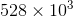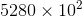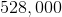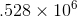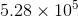Explanation:

100 miles = 528,000 feet. To put a number in scientific notation, we put a decimal point to the right of our first number, giving us 5.28. We then multiply by 10 to whatever power necessary to make our decimal equal the value we are looking for. For 5.28 to equal 528,000 we must multiply by 10^5.### Example Question #12 : Gre Quantitative Reasoning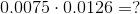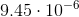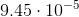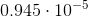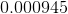Explanation:

This question requires you to have an understanding of scientific notation. Begin by multiplying the two numbers: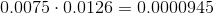To use scientific notation, the number to the left of the decimal has to be between 1 and 10. In this case, we are looking to move the decimal place until we are left with 9 on the left of the decimal. Count the number of places that the decimal will have to move. In this case, it is five. Therefore:Note: The notation is raised to a negative power because we moved the decimal from left to right.

Tired of practice problems?

Try live online GRE prep today.